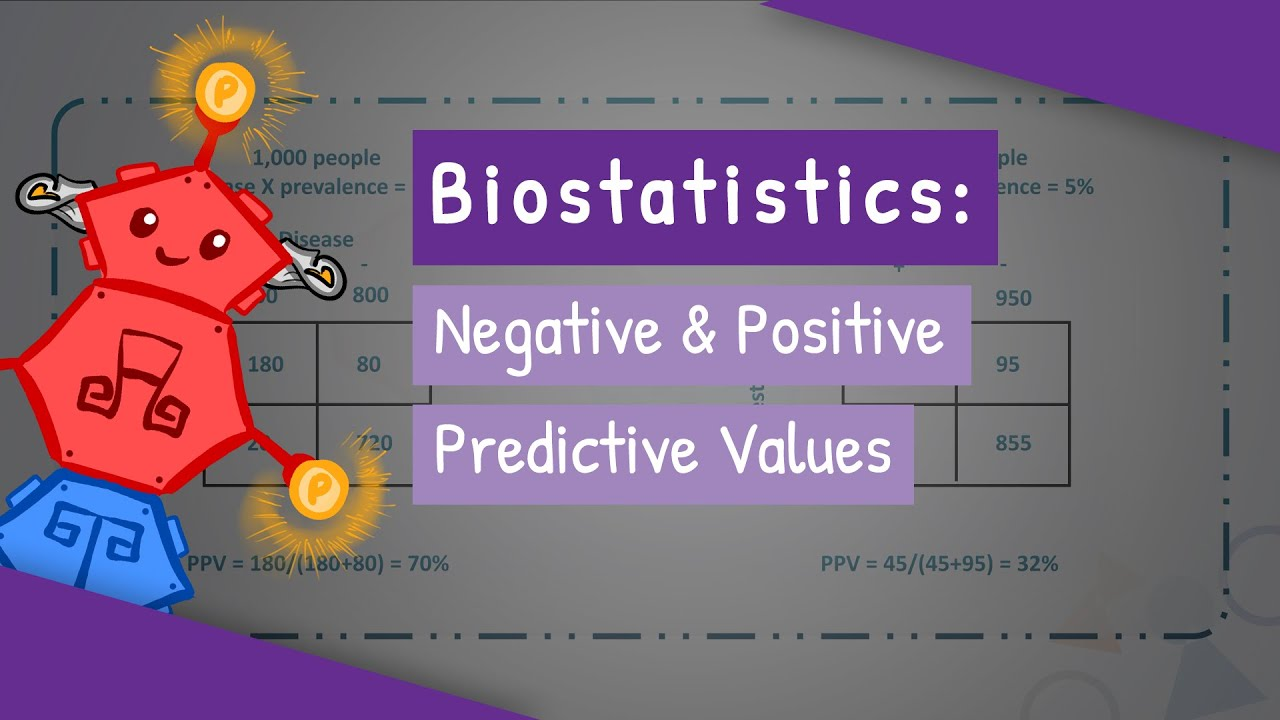# Dummies guide to Positive Predictive Value.When we take a medical diagnostic test to see if we are infected with a particular pathogen (like, say SARS-CoV-2, or HIV, or MRSA) we would ideally hope that the assay is perfect. In this perfect world, a positive test result would indicate with 100% certainty that you are infected with the pathogen, and a negative test result would indicate with 100% certainty that you are not infected.

Sadly, we don't live in that world. Assays aren't (for multiple reasons) perfect, and a "positive" test result is actually a prediction (with a computable accuracy) that the patient is infected with the relevant pathogen. In biostatistics, that computable accuracy value is called "Positive Predictive Value", or just PPV.

All diagnostic assays have two critical attributes that can be measured: sensitivity and specificity.

Sensitivity is "if the patient if actually infected, what are the chances that this assay will say so?". A typical way to measure the sensitivity of an assay is to start with a cohort of samples that we are infected, test each of them with the assay in question, and find out what percentage of samples test "positive". For instance, if we had a cohort of 100 samples that we were positive and our assay indicated that 98 of them were positive (i.e., it missed two of them), we would say that the assay has a sensitivity of .98. (A little reflection will demonstrate that means that the false-negative rate for our hypothetical assay is .02, or "1 - sensitivity")

Specificity is "if the patient is NOT actually infected, what are the chances that this assay will say they're not?" A typical way to measure the specificity of an assay is to start with a cohort of samples that we are not infected, test each of them with the assay in question, and find out what percentage of the samples test "negative". For instance, if we had a cohort of 100 samples we were negative and our assay indicates that 93 of them were negative (i.e., it misdiagnosed 7 of them), we would say the assay has a specificity of .93. Again, a little reflection will demonstrate that the rate of false positives for our hypothetical assay is .07, or "1 - specificity")

I want to highlight here that while "1 - specificity" shows the false-positive rate for the assay, it does not directly tell us what the false-positive rate will be when we're using this assay to test the general population.

Here's why:

Let's pretend our hypothetical assay has a specificity of .99. That means that the false-positive rate (computed as "1 - specificity) will be .01. Let's use this assay to test 1000 people from a population (that is, in our thought experiment, the disease we're looking for is entirely absent from the population)

In this thought experiment, .01 (i.e., 1%) of the 1000 people we test will have a positive test result (that's a total of 10 people with a positive test result)... and 100% of those positive test results will be false-positives. This thought experiment shows that in order to determine the PPV of an assay, we need to know not just the sensitivity and specificity of the assay in question, we need to know the prevalence of the pathogen within the population. [Re-read this paragraph until it's intuitive.]

With all of that in mind:

The PPV of an assay is the ratio of true positives results (henceforth called TP) to the total positive results. (Let's call the total number of positive results, TotP)

As an equation, that's PPV = TP/TotP

TotP can be broken down as "True positive results + false positive results", so let's re-write that equation:

`Equation 1: PPV = TP/(TP + FP)`

Remember when we realized that PPV depends on the prevalence of the disease in the population? Let's let "Pr" stand for prevalence (the percentage of people genuinely infected), and "Pop" stand for the number of people in the population. With those priors, the number of people in the population that are infected is "Pr x Pop", and the number of TP (true positive test results) = "Pr x Pop x Sensitivity". A little bit later, we're going to substitute "Pr x Pop x Sensitivity" into Equation 1 in both places we see a TP" there.

A little earlier, we said that we would call the prevalence of the pathogen in the population "Pr". If "Pr" is a measure of the portion of the population that is infected, then the portion of the population that is NOT infected is "1 - Pr". That means the total number of people who are NOT infected is "Pop x (1- Pr)"

Remember when we said that the "false positive" rate of an assay is "1 - Specificity"? A "FP" (false positive test result) is when one of those people who are NOT infected [i.e, "Pop x (1 - Pr)] has a test result that is positive. To compute the total number of false positive test results, we get the equation FP = (1-Specificity) x Pop x (1 - Pr).

Let's rewrite Equation 1 with our new definitions for TP and FP:

`Equation 2: PPV = (Pr x Pop x Sensitivity) / (Pr x Pop x Sensitivity) + ((1-specificity) x Pop x (1- Pr))`

Notice that Pop (the total number of people being tested) is in all three terms of that equation, so we can factor it out:

`Equation 3: PPV = (Pr x Sensitivity) / (Pr x Sensitivity + ((1 - Specificity) x (1 - Pr))`

That's it... that's how to compute PPV. Thank you for sitting through my TED talk on intro to biostatistics. 🙂

(If you're wondering whether there is a similar method for computing NPV, there is and I may get to that at a later point)